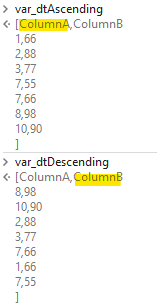# Sorting columns without sort datatable activity

Hi guys
I want to sort colum of excel without “sort datatable activity”

ColumnA should be ascending
ColumnB should be descending

How to do

1 Like

Hi

Have a view on this thread for complete referral

As you want to have it without sort activity use this expression in a assign activity

Dt = Dt.AsEnumerable().OrderBy(Function (row) Convert.ToInt32(row(“columnname”)).Select(Function (row) row).ToArray.CopyToDatatable()

For descending

Dt = Dt.AsEnumerable().OrderByDescending(Function (row) Convert.ToInt32(row(“columnname”)).Select(Function (row) row).ToArray.CopyToDatatable()

Cheers @Shoebmd

Hi @Shoebmd !

You can do the following, will leave some useful links below!

LINQ Queries “OrderBy” and “OrderByDescending”

Code (inside an assign activity)

``````var_dt.AsEnumerable.OrderBy(function(x) x("ColumnA")).CopyToDataTable
``````

Code (inside an assign activity)

``````var_dt.AsEnumerable.OrderByDescending(function(x) x("ColumnA")).CopyToDataTable
``````

This would give the following results:OrderBy Info : OrderBy - Microsoft Doc
OrderByDescending Info: OrderByDescending - Microsoft Doc

Hope this helps!

i have one excel file in that two columns are there (ColumnA) and (ColumnB) i want to sort both column simultaneously please tell me for this scenario

Fine

Have a view on this thread for more ideas on multiple column sorting

Cheers @Shoebmd

In General WE can do

Assign Activity
Lhs: dtfiltered
Rhs

(From d in yourdtvar.asenumerable
Order by d(“colA”).tostring, d(colB).toString Descending
Select r=d).copytodatable

As you Had No mentioned the colum Datatype, Maybe you have also to Concert. Bin this IS easyadopted

Writtenfrom cellohone, Just Cross hec the syntax

This is the expression that can be used

Use a assign activity and mention like this

dt = (From x In dt.AsEnumerable()
Order By x(“ColumnName-1”), x(“ColumnName-2”)
Select x).CopyToDataTable

dt is the datatable variable name

Cheers @Shoebmd

Updated above

Datataype is integer

Hi,

Try below one

(From d in InputDT.AsEnumerable
Order by CInt(d(“colB”)) Descending, CInt(d(“colA”))
Select d).Copytodatable()